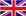English
Blog

### Winding method of current transformer

July 13 , 2020
The current transformer is an instrument that accurately converts a large amount of current on the primary side into a small amount of current on the secondary side according to the magnetic effect of the current. The current transformer is composed of a closed iron core and windings. The number of primary winding turns is very small, and the string is in the route of the current to be accurately measured; the number of secondary winding turns is relatively large, which is connected in series in the detection instrument and the protection circuit. The secondary side of the current transformer cannot be opened.

Appropriate way of winding and calculation of turns

We should first clarify the multiple of the transformer according to the load is too small, and then wind the primary wire from the center of the transformer according to the regulations. Note that the number of turns on the outside can not be used as the number of windings. The number of turns in the device is standard.

For a current transformer with a maximum converter ratio of 150 /5, its primary maximum rated current is 150 A. If it needs to be reused as a 50 /5 transformer, the transmission line should pass through 150 /50 = 3 turns, ie the inner ring Through 3 turns, there are only 2 turns on the outside. That is, you can see through a few turns, if you count the number of turns in the inner circle.

Calculation of transformation ratio and turns

That is, the current transformer transformed into 50 /5, the number of turns through the core at one time is 3 turns. Similarly, if the ratio of the original current transformer is 50/5 and the number of core-turns is 3 turns, to change it to a 75 /5 transformer application, we first measure the maximum primary rated current in the case of a single turn: Maximum primary rated current = primary current in the original application × original number of turns through the core = 50 × 3 = 150 A.

The number of through-core turns after conversion to 75 /5 is 150 /75 = 2 turns. That is, when the 50 /5 current transformer with the original number of core-turning turns of 3 turns is converted into a 75 /5 current transformer, the number of core-turning turns should be 2 turns.

Another example is the 50 /5 current transformer with 4 core turns, which needs to be converted into a 75 /5 current transformer application. We first calculate the maximum primary current of 50 × 4=200 A, and change the wear after application The number of core turns should be 00 /75 ≈2.66 turns. The number of winding turns is only an integer when the core is actually threaded, either 2 turns or 3 turns. Whether we wear 2 turns or 3 turns, there will be deviations in accurate measurements.

Therefore, when you are not clear about the maximum primary rated current of the current transformer, it is not possible to carry out variable ratio disassembly, otherwise it is very likely to cause deviations in measurement verification.

#### Tags

welcome to ZTC
Talk To ZTC Today To Discuss Your Need For Current Transformers,Get Standard Item, OEM/ODM Service Quote Are Available.